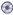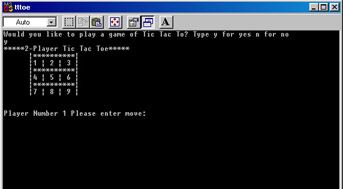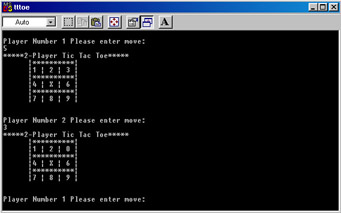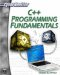# Simple Command-Line Games

Rather than discuss the various aspects of a simple game in abstract terms, it would probably be useful for us to take a look at the code for a simple game. Example 14.1 shows you a fully working, two-player Tic Tac Toe game. Before you dive into the code, it would probably be a good idea to have a basic idea of what each of the functions in the code do. Table 14.1 summarizes each function and its purpose.

Table 14.1: The Functions and Variables of the Tic Tac Toe Game

Function/Variables

Purpose

StartGame()

This function simply starts the game.

Next_Player_Moves()

This function is used after one player moves.

Move()

This function does the initial move for the game.

Check_For_Win()

This function checks the various possible winning combinations, to see if any player has won.

DrawBoard()

This function draws the board on the screen.

Board

This array is a 3 by 3 array of integers that represent the actual Tic Tac Toe board.

turn

This contains a number indicating whether player one or player two is the current player.

IsGameOver

This is an integer value representing whether the game has been won.

In addition to this table, there are extensive comments throughout the code, explaining each function and variable. This should make the code relatively easy to follow and understand. It is also important for the reader to note that the actual code consists of basic techniques that have already been covered in depth in this book. The real key to games programming comes from the creative use of these functions.

 On the CD Hint! Some of the lines of code in Example 14.1 are a bit long and may wrap to the next line. If you have trouble following those portions of code, just look at the example on the accompanying CD-ROM.

### Example 14.1

Step 1: Enter the following code into your favorite text editor and save it as14-01.h.

`#include <iostream> using namespace std; // constants to show whose turn ti is const int PLAYER1 = 1; const int PLAYER2 =2; class TicTacToe {  int board;  // 3 X 3 board to play on  int turn;// whose turn is it  int isGameOver;   // is the game over?  void PlayGame();  // start game  public:      // functions for making      // moves and starting the game      void StartGame();       void Next_Player_Moves();  int Move(int);      // other functions  void Check_For_Win();  void DrawBoard(); }; void TicTacToe::StartGame() {      // This function clears the board and starts the game for(int i = 0; i < 3; i++) for(int j = 0; j < 3; j++)  board[i][j] = 0;// A zero is indicative of an empty       // square  turn = PLAYER1;   isGameOver = 0;  DrawBoard();  // This function draws the game  PlayGame();   // This starts playing the game } int TicTacToe::Move(int i) {      // the math is all based on three's      // since each row has three choices.  int x = (i - 1)/3;  int y = ((i + 2) % 3);  int returnVal = board[x][y];  if (returnVal == 0)      {    board[x][y] = turn;    Check_For_Win();    if (!isGameOver)        {  Next_Player_Moves();        }// end of inner if       }// end of outer if   else   cout << "Invalid move, try again.\n";       DrawBoard();// Redraw the board       return returnVal; } void TicTacToe::Next_Player_Moves() {      // This function checks to see whose turn it was last      // then gives the other player a turn    if (turn == 1)  turn = PLAYER2;    else  turn = PLAYER1; } void TicTacToe::Check_For_Win() {      // This checks to see if the game is over, and who      // has won it. It does this by checking the various         // possible combinations that might lead to a win. This         // is done in a series of nested if statements checking        // each possible win scenario.  if ((board == turn) && (board == turn) &&    (board == turn))    {isGameOver = turn;}  else    if ((board == turn) && (board == turn) && (board == turn))  {isGameOver = turn;}    else if ((board == turn) && (board ==    turn) && (board == turn))   {isGameOver = turn;} else   if ((board == turn) && (board ==  turn) && (board == turn)) {isGameOver = turn;}   else if ((board == turn) && (board ==    turn) && (board == turn))   {isGameOver = turn;} else   if ((board == turn) && (board  == turn) && (board == turn)) {isGameOver = turn;}   else if ((board == turn) &&   (board == turn) && (board  == turn))   {isGameOver = turn;} else    if ((board == turn) &&  (board == turn) && (board == turn))  isGameOver = turn; } void TicTacToe::PlayGame() {   int imove;      // if the game is not over (i.e. if isGameOver!= turn)       // then prompt for the next move. while (isGameOver!=turn)      {    //DrawBoard();    cout << "Player Number " << turn << " Please enter    move: \n";    cin >> imove;    Move(imove); }      // when a player wins the while loop will stop      // and we can display the winner cout << "Player Number " << turn << "  Wins!" << endl; } void TicTacToe::DrawBoard() {      // this function simply draws the      // Tic Tac Toe board.      int temp; int k = 0; for(int i = 0; i < 3; i++) for(int j = 0; j < 3; j++)      {    if (board[i][j] == 0)  temp[k] = k+49;    else      {   if (board[i][j] == 1) temp[k] = 88;   // this creates an x   else temp[k] = 79;   // this creates a o    }    k++;// end of outer if  }     // end of inner for loop      cout << "*****2-Player Tic Tac Toe*****\n";  cout << " |**********|\n";  cout <<" |" << (char)temp << " | " <<    (char)temp << " | " << (char)temp << " | \n"; cout << " |**********|\n"; cout <<" |" << (char)temp << " | " << (char)temp << " | " << (char)temp << " | \n"; cout << " |**********|\n"; cout <<" |" << (char)temp << " | " <<    (char)temp << " | " << (char)temp << " | \n"; cout << endl << endl; }`

Step 2: Enter this code into a text editor and save it as14-01.cpp.

`#include "14-01.h" #include <iostream> using namespace std; // prototype the menu function void menu(); // create an instance of the Tic Tac Toe class TicTacToe mygame; int main() {    // the menu is in a separate function thus making     // it easier to repeatedly call it, allowing you to keep     // playing Tic Tac Toe.        menu();    return 0; } void menu() {    char choice; // this finds out it the user wisher  // to to continue or not.       cout << "Would you like to play a game of Tic Tac To?"   cout << "Type y for yes n for no\n";      cin.get(choice);      if (choice=='y') {        mygame.StartGame();        menu();      }      else      {        return;      } }`

Step 3: When you run the program, you will see images like those shown in Figures 14.1 and 14.2.Figure 14.1: Intro to the Tic Tac Toe game.Figure 14.2: Playing the Tic Tac Toe game.

In this game, we used basic code and a rather simple class structure to create an interactive two-player game. The actual flow of this game is fairly straightforward and is depicted in Figure 14.3.Figure 14.3: The flow of the Tic Tac Toe game.

The flow starts with the int main function, as all C++ programs begin. Main then calls the function menu, which will call the StartGame function of the TicTacToe class. The StartGame function is, of course, the starting point for the Tic Tac Toe game. It calls the DrawBoard function that draws the actual 3 by 3 Tic Tac Toe game board, and then calls the move function, that makes the very first move. After that move, the Next_Player_Moves function is called. That move function will continually call the Check_For_Win function. If no one has yet won, then the Next_Player_Moves function is called. This loop is the key to keeping the game moving. Recall that it was mentioned earlier that loops are critical in games programming. And, of course, the Check_For_Win function is based entirely on a series of nested if statements that check to see if any winning condition for the game has been met.C++ Programming Fundamentals (Cyberrookies)
ISBN: 1584502371
EAN: 2147483647
Year: 2005
Pages: 197
Authors: Chuck Easttom